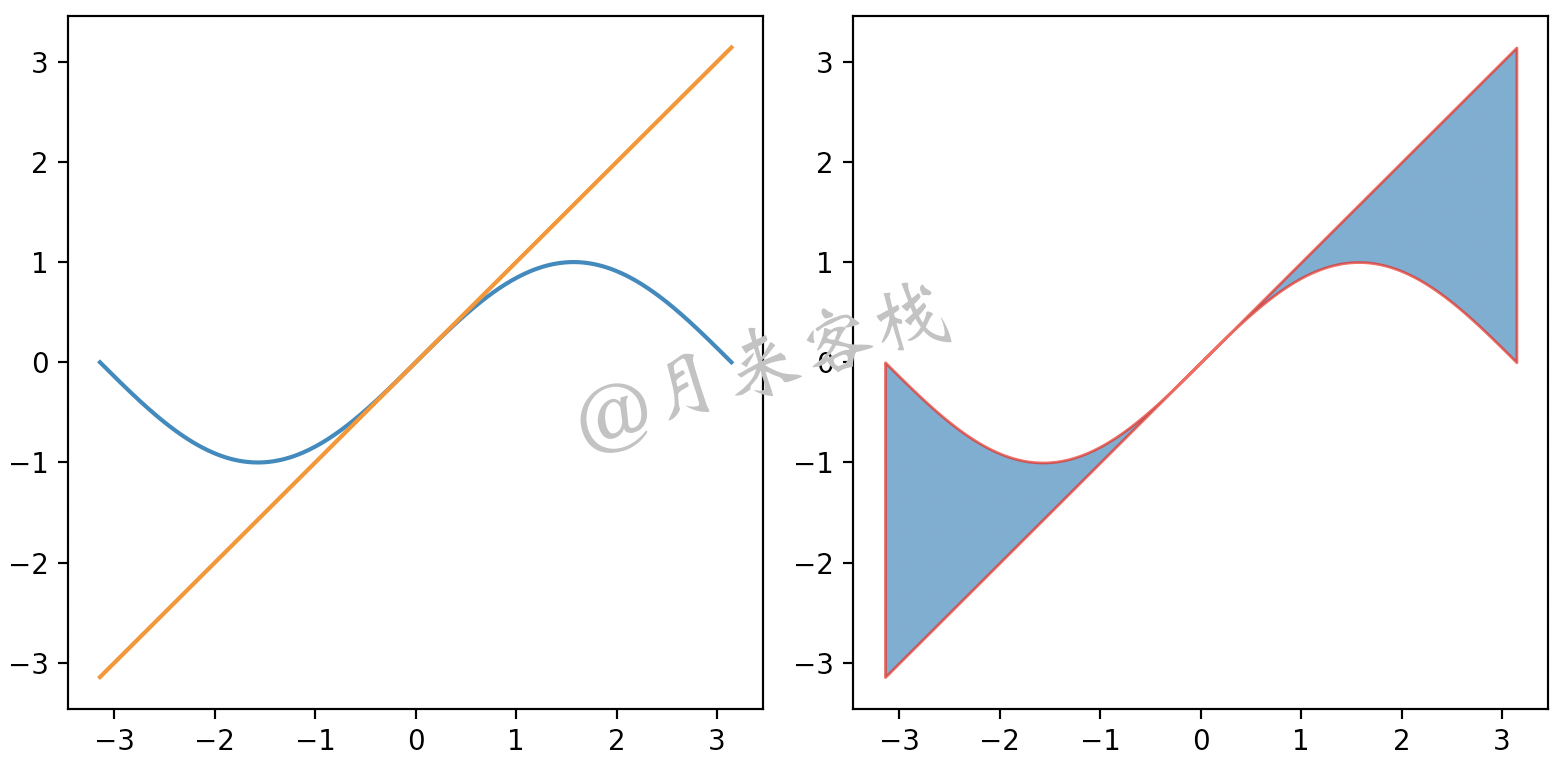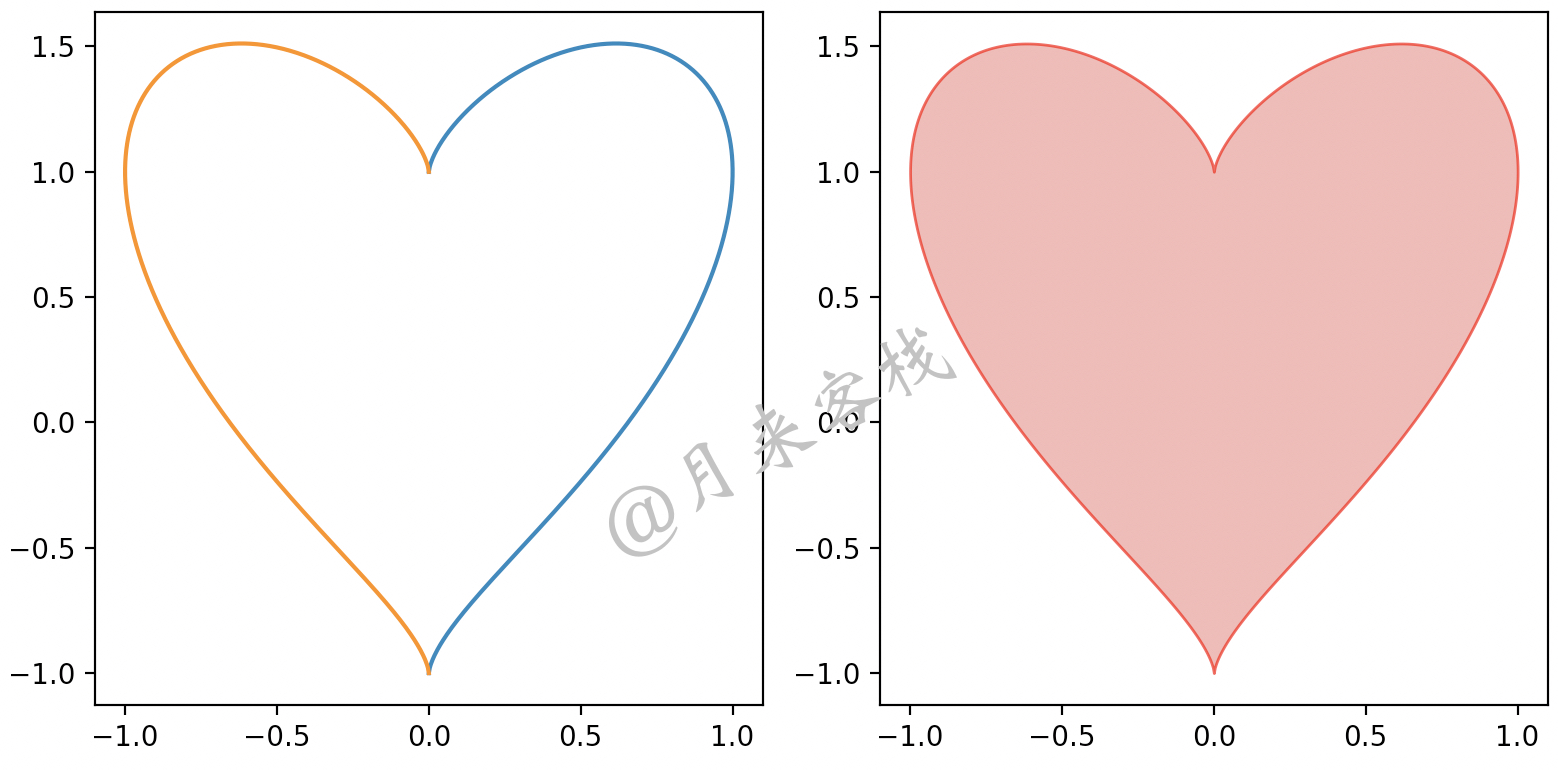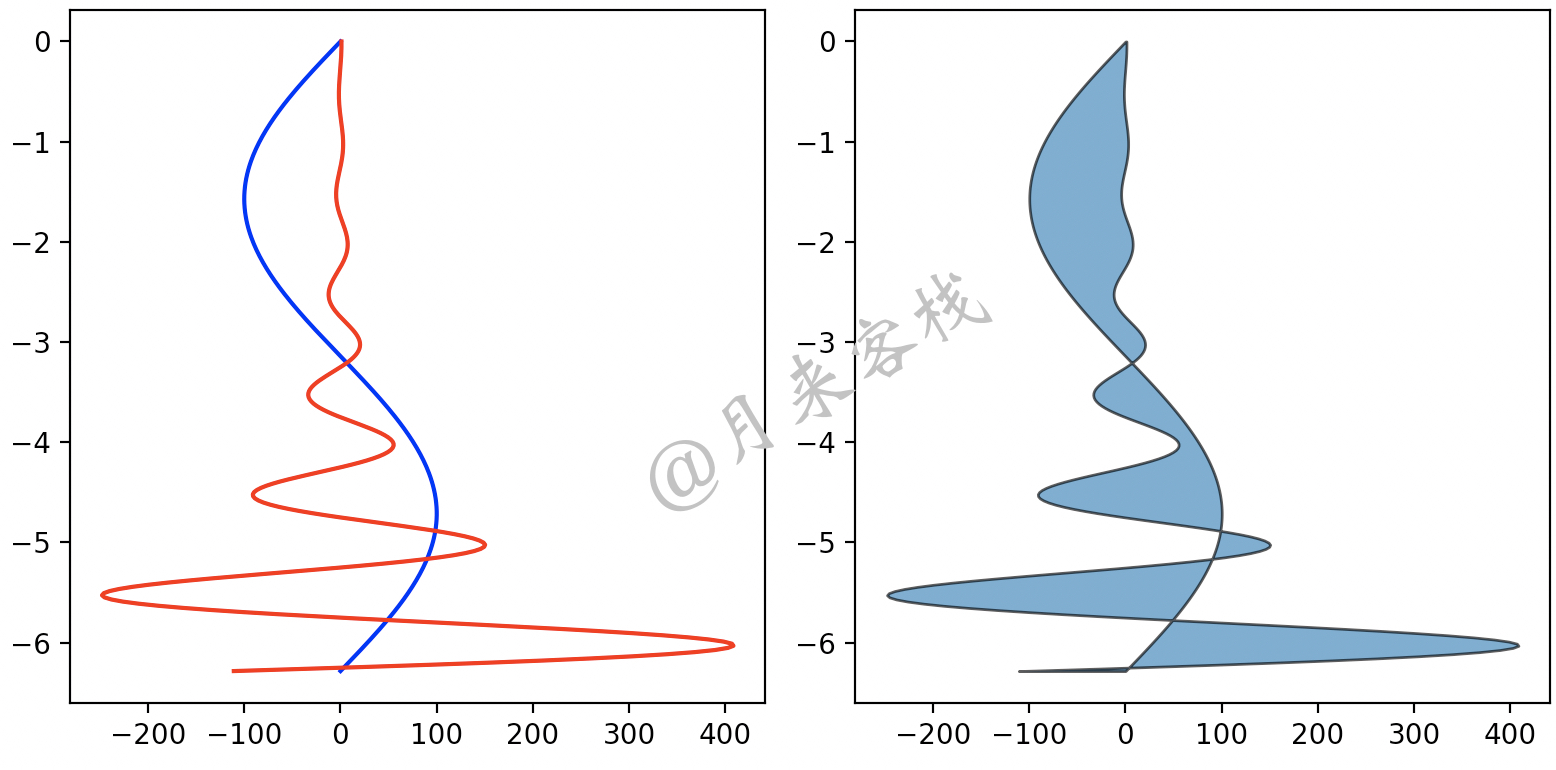### 1 引言### 2 填充图

#### 2.1 fill_between方法

1def fill_between(x, y1, y2=0,edgecolor=None, facecolor=None, alpha=None):2    pass

xxxxxxxxxx121def plot2():2    plt.figure(figsize=(8, 4))3    x = np.linspace(-np.pi, np.pi, 300)4    y1 = np.sin(x)5    y2 = x6    plt.subplot(1, 2, 1)7    plt.plot(x, y1)8    plt.plot(x, y2)9    plt.subplot(1, 2, 2)10    plt.fill_between(x,  y1,y2, edgecolor='red', facecolor = 'blue',alpha=0.7)11    plt.tight_layout()12    plt.show()

#### 2.2 fill_betweenx方法

xxxxxxxxxx21def fill_betweenx(y, x1, x2=0, edgecolor=None, facecolor=None, alpha=None):2    pass

xxxxxxxxxx121def plot3():2    plt.figure(figsize=(8, 4))3    y = np.linspace(-2 * np.pi, 0, 300)4    x1 = 100*np.sin(y)5    x2 = np.cos(2 * np.pi * y) * np.exp(-y)6    plt.subplot(1, 2, 1)7    plt.plot(x1, y, c='blue')8    plt.plot(x2, y, c='red')9    plt.subplot(1, 2, 2)10    plt.fill_betweenx(y, x1, x2, edgecolor='black', alpha=0.7)11    plt.tight_layout()12    plt.show()xxxxxxxxxx141def plot4():2    plt.figure(figsize=(8, 4))3    t = np.linspace(0, np.pi, 1000)4    y = np.cos(t) + np.power(np.sin(t), 2.0 / 3) 5    x1 = np.sin(t)6    x2 = -np.sin(t)7    plt.subplot(1, 2, 1)8    plt.plot(x1, y)9    plt.plot(x2, y)10    plt.subplot(1, 2, 2)11    plt.fill_betweenx(y, x1, x2,edgecolor='red', 12                      facecolor='#ef8683', alpha=0.7)13    plt.tight_layout()14    plt.show()